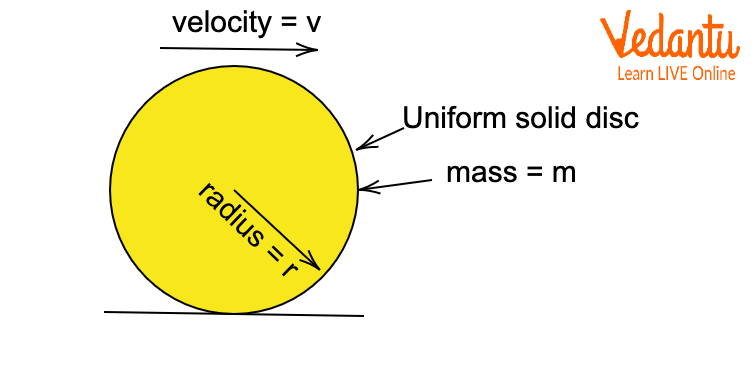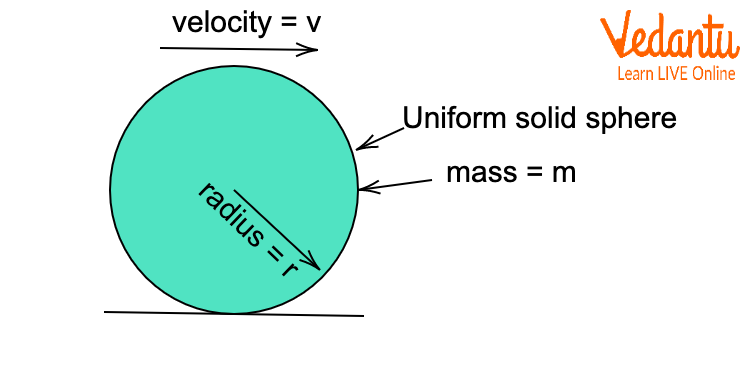Courses
Courses for Kids
Free study material
Offline Centres
More

# Uniform Pure Rolling - Definitions, Concepts, and Formulas for JEELast updated date: 02nd Dec 2023
Total views: 22.5k
Views today: 0.22k## What is Rolling Motion?

Every rigid object can exhibit two different types of motion that are translational motion and rotational motion. Translational motion is a condition when there is the displacement of the object in a given interval of time. The rotational motion of an object is a condition when there is an angular displacement of the object in a given interval of time. There are conditions when an object translates and rotates around an axis simultaneously. Rolling is one example of these types of motion. Rolling is a combination of translational and rotational motion. The centre of mass of a rolling body translates in a direction and the body itself rotates about an axis. In this article, we will be discussing a special case of rolling, i.e., pure rolling.

## Pure Rolling

Imagine a car moving on a horizontal surface. The wheels of the car will be in a state of rolling as they are both translating and rotating at the same time. Now, imagine a sudden obstacle comes and brakes are applied, there will be skidding of wheels on the surface. Now imagine another case where a wheel goes in a patch of mud. The surface will not have enough friction and again the wheel will slip on the surface.

In both of the above cases, there is rolling but there is slipping between the wheels and the surface. This is the case of impure rolling. Impure rolling is a condition when the body is in the state of rolling but there is relative slipping between the surface and the rolling body. But a special condition where there is no slipping between surfaces or say there is rolling without slipping, this condition is called pure rolling.

When there is no slipping between the rolling body and the surface, then the point of contact of the rolling body with the surface must be at rest with respect to the surface itself and if the surface is fixed, then the point of contact will have zero velocity. Imagine a ball of radius $R$ moving with a velocity v and angular velocity $\omega$ in a pure rolling condition on a fixed surface.Pure Rolling Disk

From the figure above the point, $P$ will be the instantaneous point of contact and will be at rest according to the condition of pure rolling. Point $P$ will have two velocities, one due to the translation of the whole ball itself and the other due to the rotation of the ball.

Velocity imparted to $P$ due to translation $=\text{ }v$

Velocity imparted to $P$ due to rotation $=R\omega$

Note that two velocities have opposite directions, so they must be equal in magnitude to cancel out each other.

$v=R\omega$

This is the necessary condition for pure rolling.

If we differentiate this equation with respect to time, we get,

$\dfrac{dv}{dt}=R.\dfrac{d\omega }{dt}$

And further, we can write

$a=R.\alpha$

where $a$ is linear acceleration and $\alpha$ is angular acceleration.

## Uniform Pure Rolling

Uniform pure rolling is a special case of pure rolling where linear velocity is constant. If no external force or torque is applied, the body will remain in a state of uniform pure rolling. The friction force for pure rolling is always zero, so there will not be any effect of the frictional force on the body.

## Kinetic Energy for a Body under Pure Rolling Motion

For a pure rolling body, it will have kinetic energy both due to translational motion and rotational motion. If there is a body of mass m, the moment of inertia $I$ around an axis moving with a velocity $v$, and angular velocity $\omega$, then

Translational kinetic energy can be written as  $\dfrac{1}{2}m{{v}^{2}}$.

Rotational kinetic energy can be written as $\dfrac{1}{2}I{{\omega }^{2}}$.

So, total kinetic energy will be equal to

$\dfrac{1}{2}m{{v}^{2}}+\dfrac{1}{2}I{{\omega }^{2}}$

## Pure Rolling for Different Round Objects

### (1) For a Uniform Ring

For a uniform ring of mass $m$ and radius $r$ and moving with a velocity $v$ under pure rolling, the moment of inertia of the ring around the perpendicular axis through the geometric centre will be $m{{r}^{2}}$. Total kinetic energy for the ring will be

$\dfrac{1}{2}m{{v}^{2}}+\dfrac{1}{2}I{{\omega }^{2}}$

Substituting I and $\omega$, and we get

$\dfrac{1}{2}m{{v}^{2}}+\dfrac{1}{2}m{{v}^{2}}=m{{v}^{2}}\text{ }\!\![\!\!\text{ }v=R\omega ]$Uniform Ring

### (2) For a Uniform Solid Disc

For a uniform solid disc of mass $m$ and radius $r$ and moving with a velocity $v$ under pure rolling, the moment of inertia of the ring around the perpendicular axis through the geometric centre will be $\dfrac{1}{2}m{{r}^{2}}$. Total kinetic energy for the ring will be

$\dfrac{1}{2}m{{v}^{2}}+\dfrac{1}{2}I{{\omega }^{2}}$

Substituting I and $\omega$, we get

$\dfrac{1}{2}m{{v}^{2}}+\dfrac{1}{4}m{{v}^{2}}=\dfrac{3}{4}m{{v}^{2}}\text{ }\!\![\!\!\text{ v=R}\omega \text{ }\!\!]\!\!\text{ }$Uniform Solid Disc

### (3) For a Uniform Hollow Sphere

For a uniform hollow sphere of mass $m$ and radius $r$and moving with a velocity $v$ under pure rolling, the moment of inertia of the ring around the perpendicular axis through the geometric centre will be $\dfrac{2}{3}m{{r}^{2}}$. Total kinetic energy for the ring will be

$\dfrac{1}{2}m{{v}^{2}}+\dfrac{1}{2}I{{\omega }^{2}}$

Substituting I and $\omega$, we get

$\dfrac{1}{2}m{{v}^{2}}+\dfrac{2}{6}m{{v}^{2}}=\dfrac{5}{6}m{{v}^{2}}\text{ }[v=R\omega ]$Uniform Hollow Sphere

### (4) For a Uniform Solid Sphere

For a uniform solid sphere of mass $m$ and radius $r$ and moving with a velocity $v$ under pure rolling, the moment of inertia of the ring around the perpendicular axis through the geometric centre will be $\dfrac{2}{3}m{{r}^{2}}$. Total kinetic energy for the ring will be

$\dfrac{1}{2}m{{v}^{2}}+\dfrac{1}{2}I{{\omega }^{2}}$

Substituting I and $\omega$, we get

$\dfrac{1}{2}m{{v}^{2}}+\dfrac{2}{10}m{{v}^{2}}=\dfrac{7}{10}m{{v}^{2}}\text{ }[v=R\omega ]$Uniform Solid Sphere

## Conclusion

Pure rolling is a combination of rotational and translational motion. When a body is pure rolling, the friction force does not have any effect on the body. So, ideally there is no energy loss due to friction, no matter how rough the surface is. This is an important characteristic feature of rolling. Rolling motion has at least energy loss, so the wheels are made round and ball bearings are used to reduce energy loss in machines. Thus, pure rolling is an important concept of rigid body dynamics which has many important applications in many areas.

## FAQs on Uniform Pure Rolling - Definitions, Concepts, and Formulas for JEE

1. What is the difference between pure rolling and rolling?

Pure rolling is a special case of rolling. In pure rolling, there is no slipping between the body and the surface and the linear velocity of the body is the product of angular velocity of the body with the radius. But in the case of rolling, slipping does exist. Take the example of the tires of an aeroplane. While landing, when the tires just touch the ground, we notice steam due to the friction, that is rolling. And when after some time, the tire starts rolling without any steam, it is pure rolling.

2. Which friction acts in the case of pure rolling?

In the case of pure rolling, static friction acts between the body and surface (F (frictional force) <=uN). This is because the point of contact during a pure roll is at rest or has no relative motion with respect to the ground (in case the ground is moving). Thus, energy remains conserved in initial and final states as frictional work is zero. In the case of rolling with slipping, there exists relative motion between body and ground as it rotates at a higher rate than it translates, that's why it slips (another condition would be skidding). And whenever relative motion prevails, kinetic friction starts acting and thus, energy is no longer conserved in initial and final states due to frictional loss.

3. Can there be pure rolling if the surface is not fixed?

Yes, as long as the instantaneous point of contact of the rolling body is at rest with respect to the surface, pure rolling will be there even if the surface is fixed or not. Take the example of the wheels of a tank. The tank has plates that roll over the wheels. In this case, the wheels are stationary but the plates or the surface moves, that is, the surface is not fixed. But still there is pure rolling in this case as there is no slipping of the plate or the wheels.